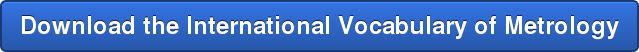## The Calibration Bench | Blog

What is Metrology?

Simply put, Metrology is the science of measurement. This is the science behind calibration.

As with any discipline, Metrology has its own unique language. This can be even more confusing as calibration occurs all over the world.

BIPM- The Internationa Bureau of Weights and Measures- created The International Vocabulary of Metrology (VIM)  to provide a common language of measurement science across all borders.

Regardless of your country of origin, we know the difference between a circle, square and rectangle. It may be called different names in different countries, but the mathematical definition is the same. The VIM takes this one step further so we can call the same things by the same name.

The goal of the VIM is to provide a standard nomenclature so we can all communicate regardless of geographical borders. Several keys concepts are defined:

• The 7 base SI units from which all others are derived
• Length (Meter)
• Mass (Kilogram)
• Time (Second)
• Electric Current (Ampere)
• Thermodynamic Temperature (Kelvin)
• Amount of Substance (Mole)
• Luminous Intensity (Candela)

• The symbols of the 7 base units
• Meter= m
• Kilogram= kg
• Second= s
• Ampere= A
• Kelvin= K
• Mole= mol
• Candela= cd

• SI PrefixesAccuracy and Precision: What do they really mean?

Definitions

measurement accuracy:

closeness of agreement between a measured quantity value and a true quantity value of a measurand

measurement precision:

closeness of agreement between indications or measured quantity values obtained by replicate measurements on the same or similar objects under specified conditions

Sometimes a Picture does a better job explaining!

Imagine a tape measure that has exactly 1.000 inch cut off of it.You will get a  precise measurement every time you use it. The problem is the measurement is not accurate.